# Solution assignment 04 Unit circle and simple formulas

### Assignment 4

Show using the unit circle: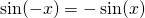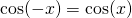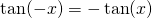### Solution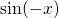has a negative projection on the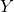-axis equally large as the positive projection of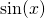on the-axis.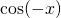has a positive projection on the-axis equally large and having the same sign as the projection ofon the-axis.
For the third formula we use the following definition of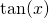in combination with some formulas concerningand: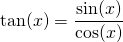Thus: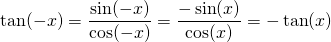0